# Kouzoujian Splitter

Click here to go to our main page on couplers and splitters

Click here to go to our page on the Lim-Eom three-way splitter

New for October 2008! This splitter came about as a way to create a three-way splitter that could be used in a planar three-way combiner. It's an alternative that Jack proposed to using the three-way Lim-Eom splitter for a power combiner. It has a huge advantage, none of the arms will be trapped inside a divider/combiner combination. Thanks again, Jack from Matrix Test Equipment!

IN a larger sense, this splitter is the answer to the question, how do I power-combine amplifiers in a planar combiner, in numbers other than 2, 4, or 8? After a message board post some initial work was done looking at using the Lim-Eom three-way splitter as a power combiner, but this particular network does not provide a convenient, phase-correct layout. This splitter we describe can combine any number of amplifiers, as opposed to purely corporate splitters which combine in binary fashion (2:1, 4:1, 8:1). It can provide equal phase/amplitude splits to each amplifier and (theoretically) infinite isolation and return losses at center frequency. We have found empirically that it also can provide 20 dB isolation over 40% bandwidth.

Because Jack thought of the idea, we encourage you to refer to this type of network as the "Kouzoujian splitter" when you reference it. Jack's sponsored page on distortion is located here.

### Three-way splitter based on unequal-split Wilkinson

The first splitter developed was a three-way, based on Wilkinson splitter, using unequal split. The network starts off as a splitter that splits 2/3 power to one port, and 1/3 to the other. Our on-line calculator quickly calculates the impedances for a fifty ohm system: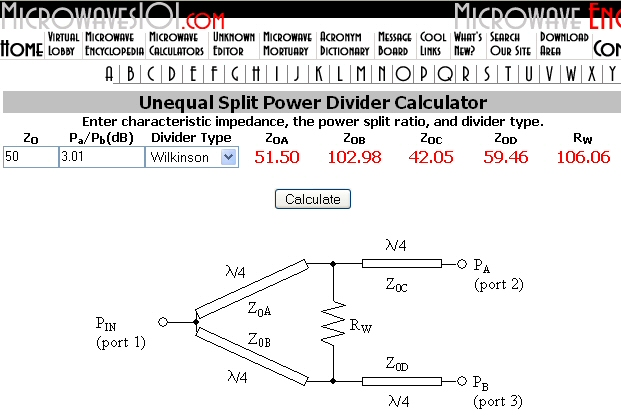This network and its RF response are plotted below: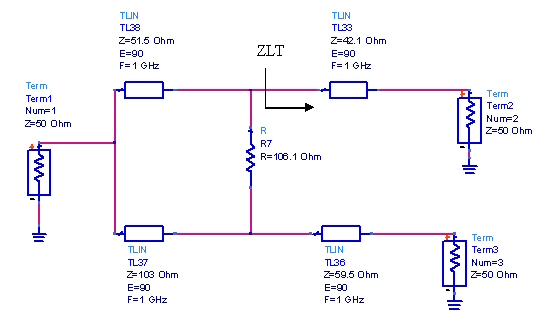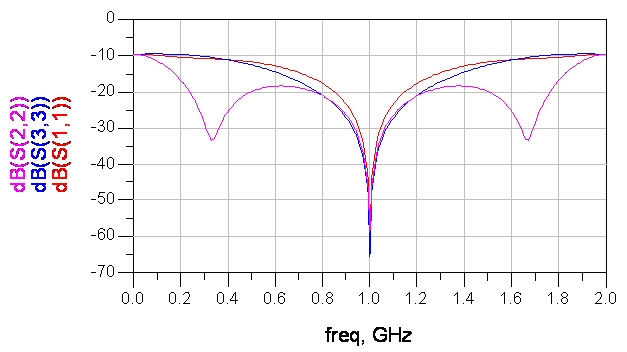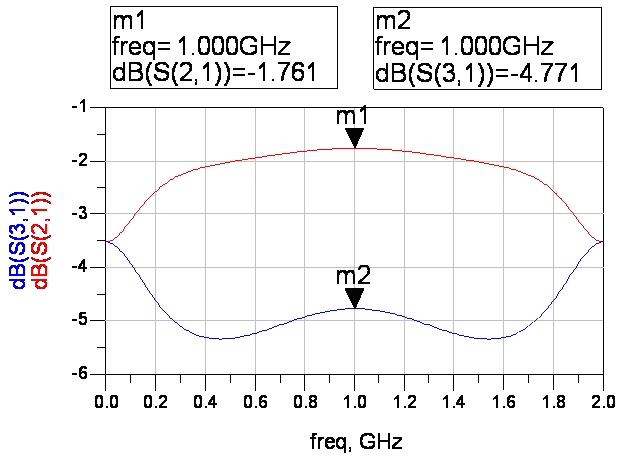The next step in creating a three-way equal splitter is to split the "favored" 66.7% split arm into two ports (an equal-split that cascades with the original unequal). Initially we can ignore the need for a second isolation resistor and just concentrate on getting the network to provide impedance match at port 1 (S11) at the center frequency. The way to do this is to determine what that port sees prior to the output transformer (impedance ZLT in the schematic), then create a second equal-split two-way splitter that will provide the necessary load. The math is simple. ZLT is found from simple quarter-wave transformer theory and is calculated as:

ZLT=ZT^2/ZL

ZT is the impedance of the transformer, and ZLT is the transformed load impedance of ZL. In this case, ZL is 50 ohms, and ZT is 42.1 ohms, so ZLT is required to be 35.4 ohms.

Next we need to transform 25 ohms (the two output ports in parallel) to this impedance. Working the same equation inside out we find that in this case ZT needs to be 29.7 ohms, however the second splitter's arm impedances are doubled to 59.5 ohms because there are two arms. The resulting three-port splitter network is shown in Figure 2. The final step is empirical, the isolation resistors are added and optimized to match the output ports. This can be done by hand tuning or with an optimization function. The resistors have no effect on the common-port impedance match (S11).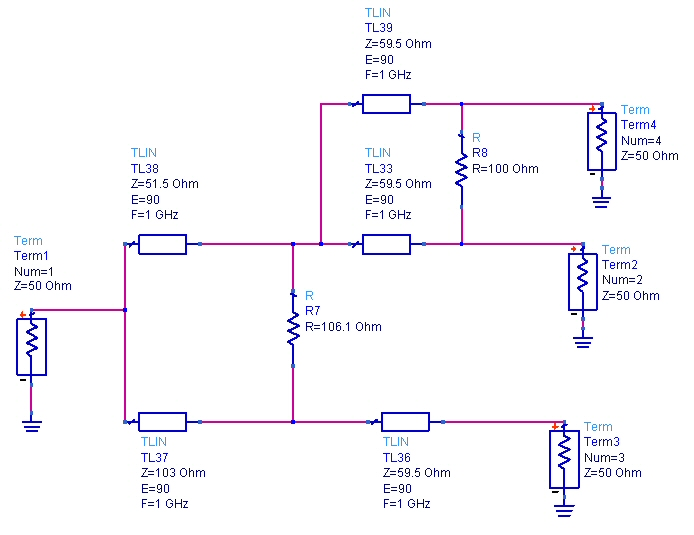Transmission, isolations and return losses: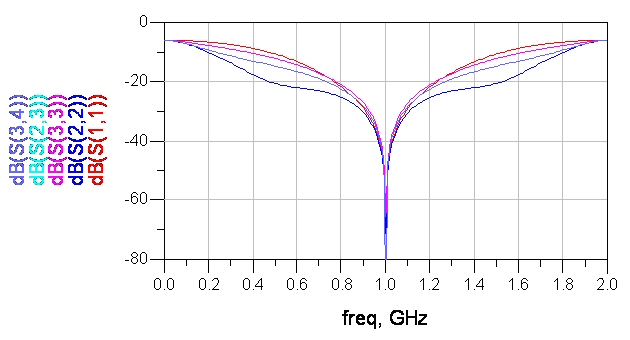### Three-way splitter based on impedance transformer

It turns out for this simple splitter there are infinite solutions to the arm impedances to achieve the center frequency perfect response, but the choice of impedances will have some effect on the bandwidth of the isolation and return losses. An alternative way to calculate the impedances is to use transformer theory. Again, the isolation resistors are ignored until the line impedances are solved to give perfect match at the common port.

In order to match three 50 ohms loads to one fifty ohm input, we need to transform them from 50 ohms to 150 ohms. In our download area there is a convenient spreadsheet that does transformer calculations. Download "the Professor's" transformer spreadsheet. Or you could spend a week making your own transformer calculator, because none of the popular EDA software packages come with this feature!

A minimum of two transformer sections are needed as shown in the previous example. We looked at equal-ripple Chebychev and maximally flat transformations, and found max-flat to provide better isolation bandwidth. Shown below is the two-section, three-arm transformer and its resulting perfect input match (S11) at center frequency.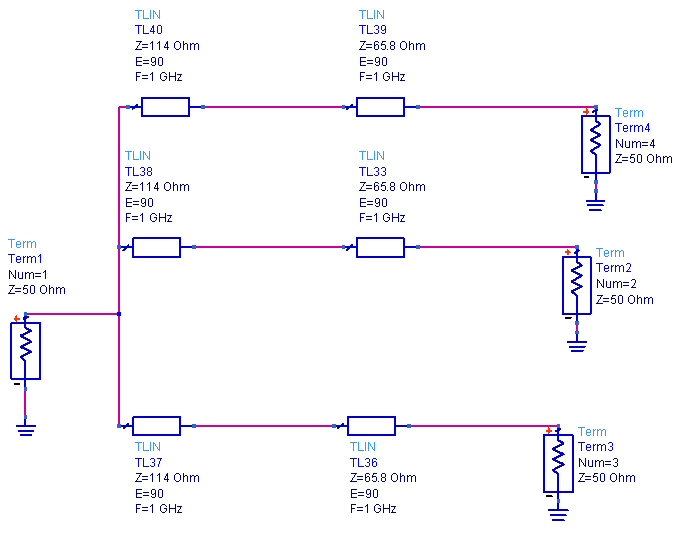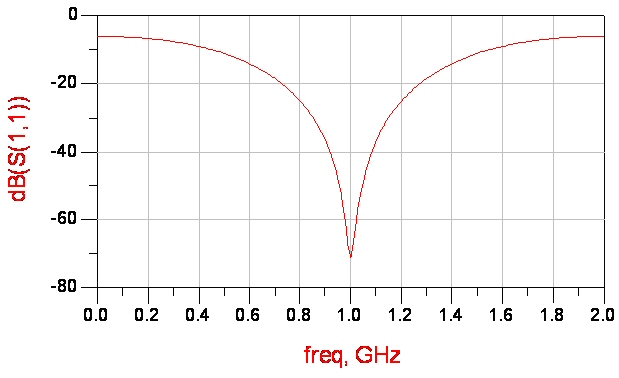Next we combine two of the arms in the first transformer stage (left side) so that we have only three-way junctions (the point is we want to avoid four-port junctions). This is done easily, the combined quarter-wave arm has 1/2 the impedance of the original.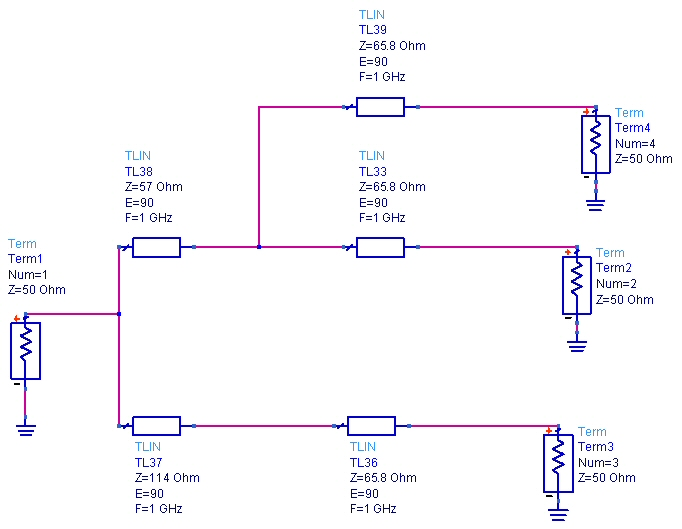There's no need to post a plot of the input match, it is unaffected by this modification.

Then next step is to add the isolation resistors. Again, this can be done empirically by tuning or with an optimizer. 100 ohms is usually a good starting value. The final network is shown below: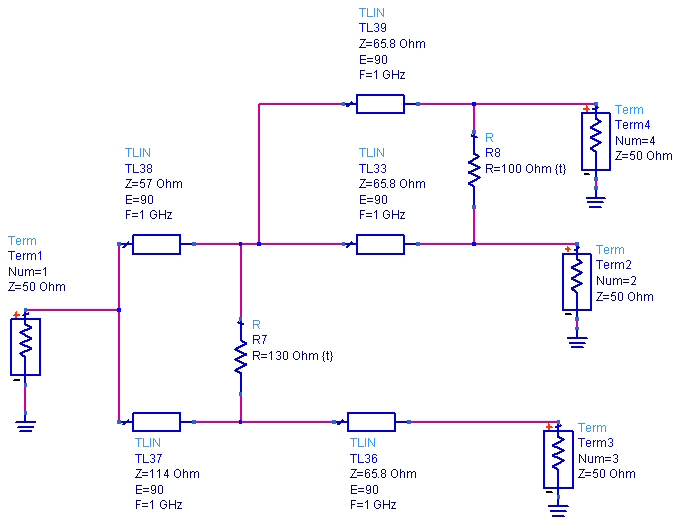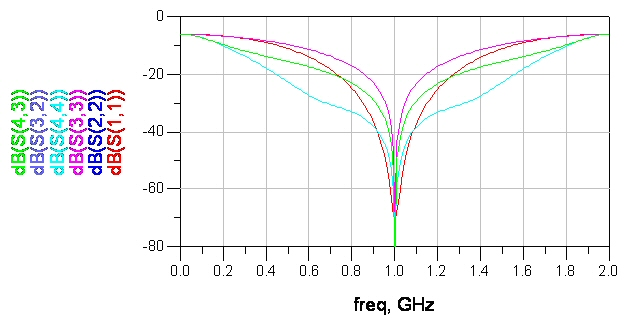Compared to the original 3-way splitter, the match on port 3 is not as wideband, but isolation is wider. We leave it up to you if you want to try using an optimizer on the network; you can get more bandwidth be slightly compromising the perfect response at the center frequency. Another trick you might find useful is to add a transformer on the input (ZT>50 ohms) which will reduce the transformer ratio needed in the splitters arms. We'll show you how this works in the next example.

More to come!

### Five-way splitter #2

In this example, we will use transformer theory to arrive at a five-way splitter network. We'll also show you how to add extra transformer sections to assist in laying out the circuit.

For an N-way split, first, determine the number of devices you want to combine (N). In the example, N=5.

Nest, determine the impedance steps for a maximally flat transformer that transforms NxZ0 to Z0. In the case of 5-way, the transformer goes from 250 to 50 ohms. 250 is the correct number because five 250 loads in parallel provide a match to 50 ohms. The resulting impedances are 242.8, 207.2, 142, 88, 60.3 and 51.5 ohms. Don't bother with Chebychev transformers, for some reason the maximally-flat transformer provides the best isolation.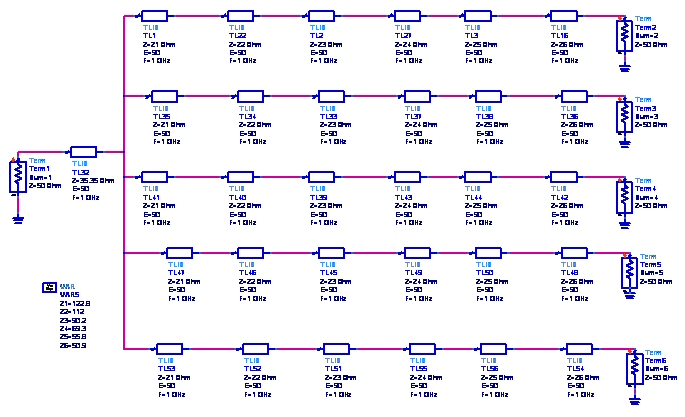In some applications, particularly at millimeter-wave frequencies you might want to add a couple of extra transformer sections to facilitate layout. For the example 5-way, we used a six section transformer, but you could achieve a mathematically-correct splitter with four sections. Using the Professor's transformer spreadsheet, we get 245.6, 224, 180.4, 138.6, 11.6 and 101.8 ohms. These are some very high impedances and will be difficult to realize in microstrip. Also, power handling is reduced when impedance is increased.

We added a single transformer on the common port that relieves the 250 ohm requirement down to 125 ohms. This is a 35 ohm transformer on the input (TL32 in the schematic in Figure 7). Now the maximally-flat transformer impedances are 122.8, 112, 90.2, 69.3, 55.8 and 50.9 ohms.
Next, using an EDA tool of your choice create a network of the splitter that starts with one node and splits out to N. Observe the response that is typical of a reactive splitter, the common port is matched but isolations and other port return losses are poor: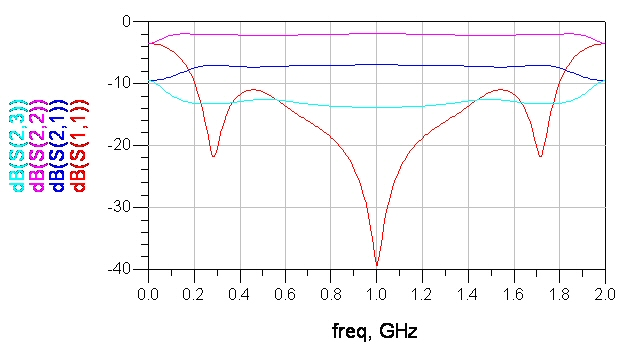The next step is to start combining some of the arms to arrive at a network that only has three-way junctions (the original network has one six-way junction we need to get rid of it). The choice of arms that you combine will be driven by layout, we want to leave some straight sections between the actual Wilkinsons. You will use unequal splits wherever you need to. See schematic. You don't need to do any calculations for the unequal splits, they will appear as required if you follow the next step. No need to consider isolation resistors at this point.

For splitter section impedances, the rule that must be followed is that the ideal transformer impedances are divided by the number of ports that they feed. Thus TL1 in the schematic is Z1/2 because it ultimately feeds two arms. TL3 is Z1/3 because it feeds three ports.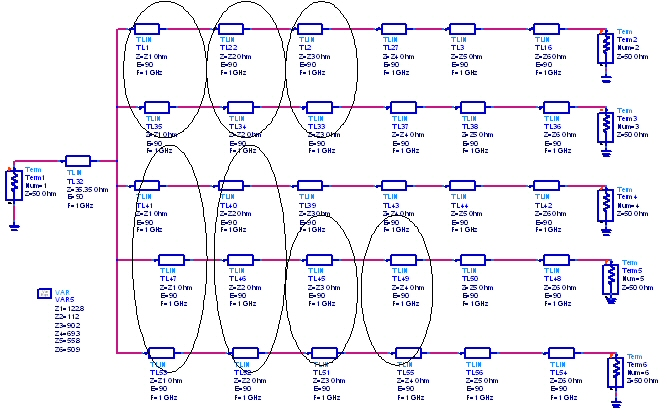The isolation resistors are located wherever it is convenient in the layout, but you must have one resistor isolation each adjacent path. The resistor values are obtained by optimization for maximum isolation and output return losses. They don't affect S11. If S11 is poor, you probably made some errors in steps 5 and 6. Combine these lines to get the impedance in the next schematic. We added the isolation resistors, again these were solved by trial and error. We still have one transmission line at 121 ohms, if this were a real design we'd push that impedance down. Remember there are infinite solutions to the arm impedances.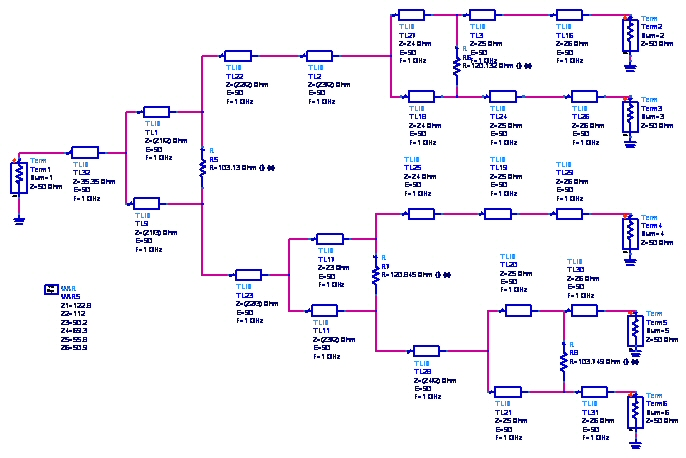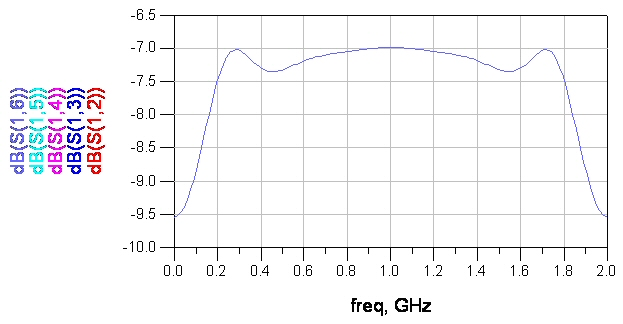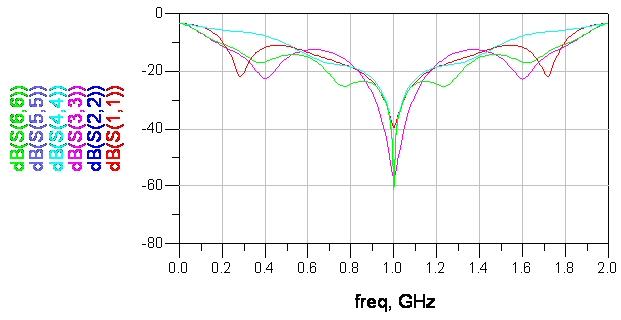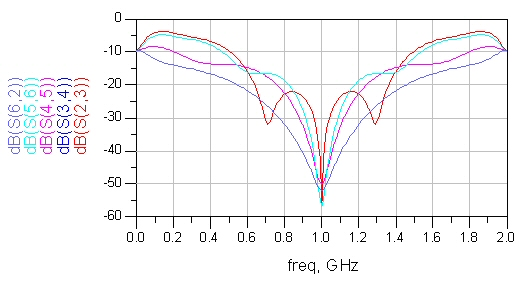In the figure below we show how the circuit might be laid our in order to maintain the correct phase relationships which are of paramount importance. In the sketch, no consideration was made to show the relative line widths to achieve the correct impedances.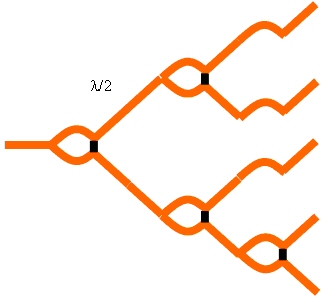### Conclusions

We have presented a new form of power splitter based on the Wilkinson hat is capable of equal amplitude, equal phase splits in theoretically any multiple.

Author : Unknown Editor